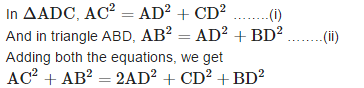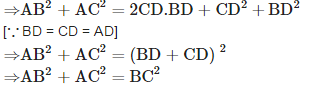Courses

# Test: Triangles- 4

## 25 Questions MCQ Test UPSC Prelims Paper 2 CSAT - Quant, Verbal & Decision Making | Test: Triangles- 4

Description
This mock test of Test: Triangles- 4 for UPSC helps you for every UPSC entrance exam. This contains 25 Multiple Choice Questions for UPSC Test: Triangles- 4 (mcq) to study with solutions a complete question bank. The solved questions answers in this Test: Triangles- 4 quiz give you a good mix of easy questions and tough questions. UPSC students definitely take this Test: Triangles- 4 exercise for a better result in the exam. You can find other Test: Triangles- 4 extra questions, long questions & short questions for UPSC on EduRev as well by searching above.
QUESTION: 1

Solution:
QUESTION: 2

### In the given figureΔABO ∼ ΔDCO. IfCD = 2cm, AB = 3 cm, OC = 3.2 cm, OD = 2.4 cm, then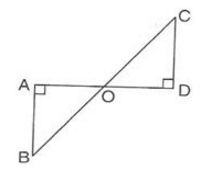Solution: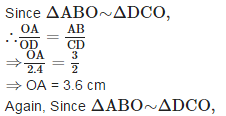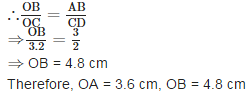QUESTION: 3

### In the given figure, AB || DE and BD || EF. Then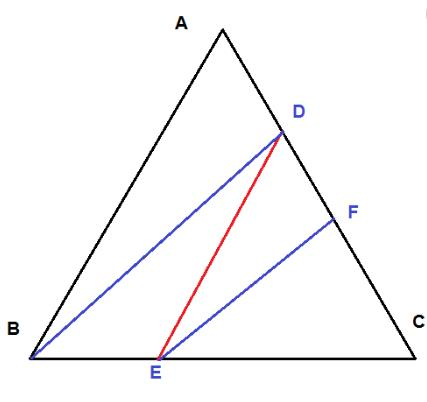Solution: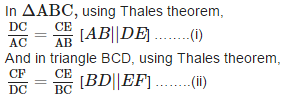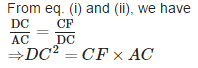QUESTION: 4

Two isosceles triangles have equal angles and their areas are in the ratio 16 : 25. Then, the ratio of their corresponding heights is

Solution:

Let two isosceles triangles be ΔABC and ΔDEF And their corresponding altitudes are AM and DN.
Since the corresponding angles of both triangles are equal.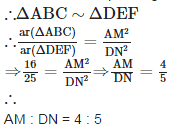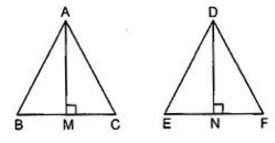QUESTION: 5

If PQR is an isosceles triangle and M is a point on QR such that PM⊥QR,then

Solution:

Since, in a triangle the sum of squares of any wo sides is equal to twice the square of half of the third side together with twice the square of the median bisecting it.
In ΔPQM,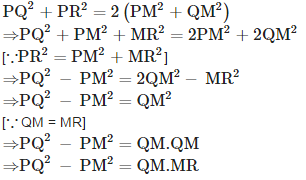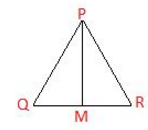QUESTION: 6

If in two triangles ABC and DEF,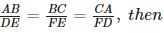Solution:

If in two triangles ABC and DEF,ΔFDE∼ΔCAB because for similarity, all sides should be in proportion.

QUESTION: 7

In trapezium ABCD, if AB||DC, AB = 9 cm, DC = 6 cm and BD = 12 cm, then BO is equal to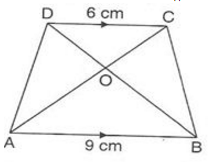Solution: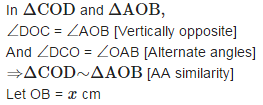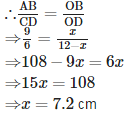QUESTION: 8

In an equilateral triangle ABC, if AD⊥BC,then

Solution:

Since the internal bisector of an angle of a triangle divides the opposite side internally in the ratio of the sides containing the angle.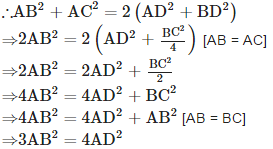QUESTION: 9

If ΔABC ∼ ΔPQR such that AB = 1.2 cm, PQ = 1.4 cm, then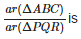Solution: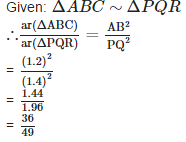QUESTION: 10

Two poles of height 8 m and 13 m are standing 12 m apart. The distance between their tops is

Solution: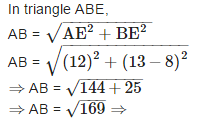AB = 13 m Therefore, the distance between their tops is 13 m.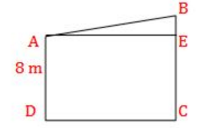QUESTION: 11

If ΔABC ∼ ΔEDF and ΔABC is not similar to ΔDEF, then which of the following is not true?

Solution:

If ΔABC ∼ ΔEDF and ΔABC is not similar to ΔDEF,ΔDEF,then BC. DE = AB .EF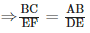Here according to given condition, BC. DE  ≠ AB .EF

QUESTION: 12

In the adjoining figure AC || BD. If AC = 6 cm, AE = 3 cm, EB = 4 cm, ED = 8 cm, then CE and BD are respectively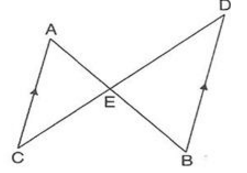Solution:

Given: AC || BD.and AC = 6 cm, AE = 3 cm, EB = 4 cm, ED = 8 cm,
In triangles ACE and DEB, ∠AEC = ∠DEB [Vertically opposite angles] ∠ECA = ∠EDB [Alternate angles as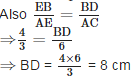QUESTION: 13

If ΔABC ∼ ΔDEF and EF = 1/3BC, then ar(ΔABC):(ΔDEF) is

Solution: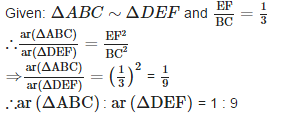QUESTION: 14

A semicircle is drawn on AC. Two chords AB and BC of length 8 cm and 6 cm respectively are drawn in the semicircle. What is the measure of the diameter of the circle?

Solution:

The diameter of circle is AC. Here ∠ABC is angle of semicircle.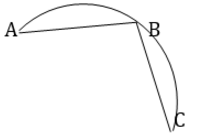AB ∴ ∠ ABC = 90° ∴ Δ ABC is aright angled triangle.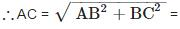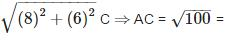10 cm

QUESTION: 15

The length of the second diagonal of a rhombus whose side is 5 cm and one of the diagonal is 8 cm is

Solution:

Let ABCD be a rhombus.
One diagonal BC = 8 cm and dise BC 5 cm
Since diagonals of a rhombus bisect each other at 90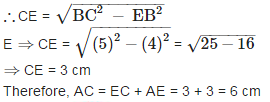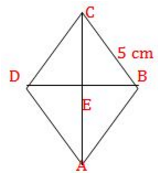QUESTION: 16

In the given figures the measures of ∠P and ∠R are respectively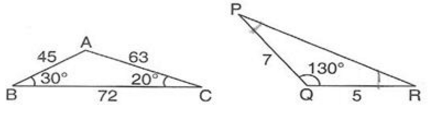Solution: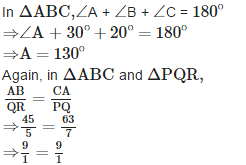Since, Sides of ΔABC and ΔPQR are proportional, therefore,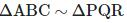.
∴ ∠A = ∠Q, ∠B = ∠R and ∠C = ∠P
⇒ ∠P
= 20° and ∠R
​= 30°

QUESTION: 17

In the given figure, AP is equal to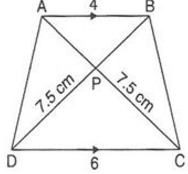Solution:

In triangles APB and CPD,
∠APB = ∠CPD [Vertically opposite angles] ∠BAP = ∠ACD [Alternate angles as AB||CD]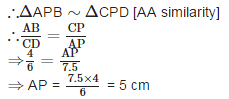QUESTION: 18

In the given figure if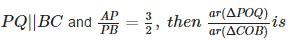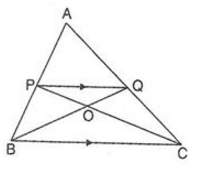Solution: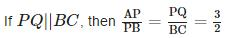In ΔPOQ and ΔCOB, ∠PQO = ∠QBO
[Alternate angles] ∠POO = ∠COB [Vertically Opposite]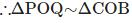.[AA similarity]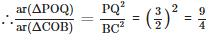QUESTION: 19

ABC is a right triangle right angled at B. BD is the altitude through B. If BD = 4 cm and AD = 3 cm then AC is equal to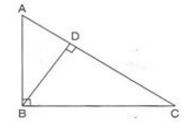Solution: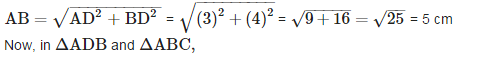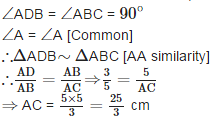QUESTION: 20

The length of the side of a rhombus whose diagonals are of lengths 24 cm and 10 cm is

Solution:

Let ABCD be the required rhombus whose diagonals are AC = 10 cm and BD = 24 cm. Let AC and BD intersect each other at O.
Then AB = BC = CD = DA
Also AO = CO = 10/2 = 5 cm
And BO = DO = 24/2 = 12 cm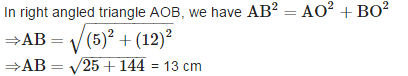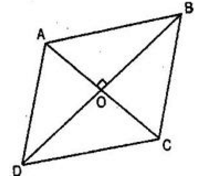QUESTION: 21

In the given figure if ∠ADE = ∠ABC,then CE is equal to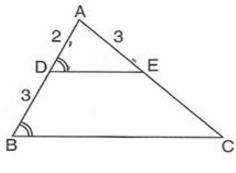Solution: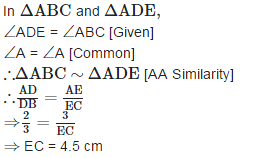QUESTION: 22

In the given figure if AT = AQ = 6, AS = 3, TS = 4, then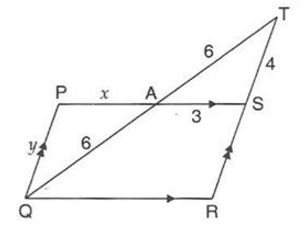Solution: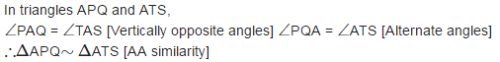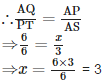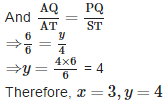QUESTION: 23

The areas of two similar triangles are 100cmand 49 cm2. If the altitude of the larger triangle is 5 cm, then the corresponding altitude of the other triangle is equal to

Solution:

Let the two similar triangles be ΔABC and ΔDEF such that ar(ΔABC) = 100 cm2 and ar(ΔDEF) = 49 cm2.
Let AM and DN be the respective altitudes of ΔABC and ΔDEF.
Since the ratio of the areas of two similar triangles is equal to the ratio of the squares of their corresponding altitudes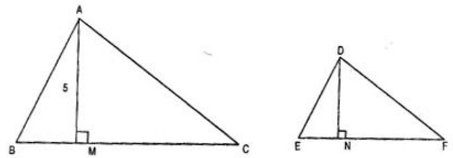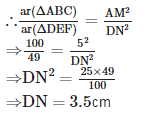QUESTION: 24

A man goes 15 m due west and then 8 m due north. How far is he from the starting point ?

Solution:

Using Pythagoras Theorem,
AC2 = AB2 + BC2 = 64 + 225 = 289 8 m ⇒ AC 17 cm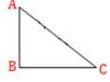QUESTION: 25

If D is a point on side BC of ΔABC such that BD = CD = AD, then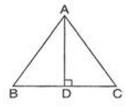Solution: